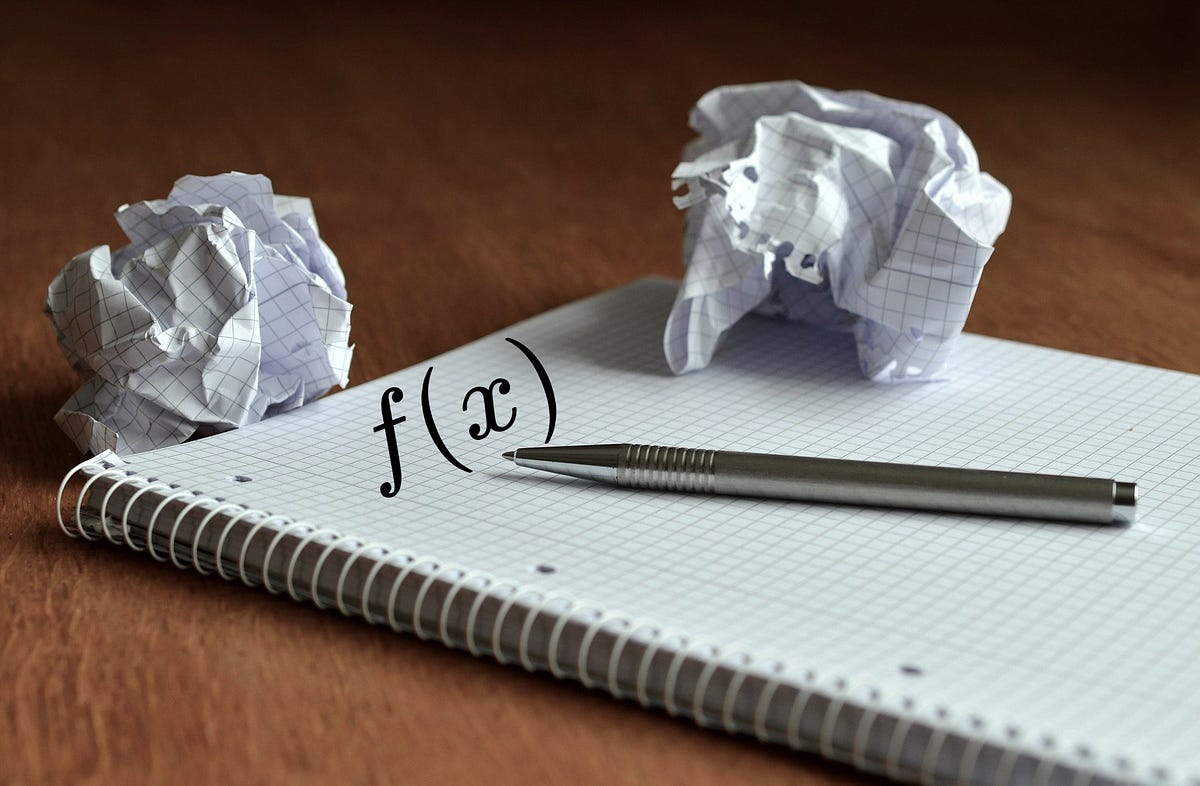# Are functions from programming really functions?Are functions from programming really functions? If you are reading this, then most probably you already know quite well what functions are in programming.

If you are reading this, then most probably you already know quite well what functions are in programming. A function is quite a common and spread programming construct that is present in almost all programming languages.

Generally, a function is a block of code that takes some parameters from outside, executes some operations in which these parameters may be used, then it returns an output value. Actually, in many programming languages functions are allowed to not return something or to return multiple values, not only one. But these cases can be also be represented, for the sake of generality, as only one value. For the “no return” case we can use a special value to represent that (in Python that special value is None; whenever you don’t return something from a function it’s returned None). And for the case of more values, we can use a vector of multiple values as a single object.

For example, in Python a function definition looks like this:

``````def func_name(param1, param2, ...):

## do some stuff with input parameters
return output_value``````

Now, the question I want to ask: Are these functions from programming true mathematical functions?

Well…, let’s first recall what a mathematical function is.

In mathematics, a function is just a mapping from a set A to a set B, in which any element from A has only one associated element in B.

## Guide to Python Programming Language

Guide to Python Programming Language

## Functional Programming: Reduce() Function in Python

Reducing sequence to a single output value! Functional Programming: Reduce() Function in Python. In the previous string of articles, I have talked about the importance of immutable data structures in functional programming. I have also covered the filter() function in the last article. Let’s start from where we left. In this article, I will be talking about the reduce() function.

## Python Programming: A Beginner’s Guide

Python is an interpreted, high-level, powerful general-purpose programming language. You may ask, Python’s a snake right? and Why is this programming language named after it?

## Python Hello World Program - Create & Run Your First Python Program in PyCharm

Python Hello World Program - Your first step towards Python world. Learn how to create the Hello World Python program in PyCharm.

## Python Programming Tutorials For Beginners

Python Programming Tutorials For Beginners